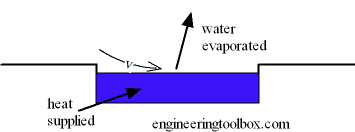Engineering ToolBox - Resources, Tools and Basic Information for Engineering and Design of Technical Applications!

# Evaporation from a Water Surface

## Evaporation of water from a water surface - like a swimming pool or an open tank - depends on water temperature, air temperature, air humidity and air velocity above the water surface - online calculator.

Evaporation of water from a water surface - like an open tank, a swimming pool or similar - depends on water temperature, air temperature, air humidity and air velocity above the water surface.The amount of evaporated water can be expressed as:

gs = Θ A (xs - x) / 3600                                 (1)

or

gh = Θ A (xs - x)

where

gs = amount of evaporated water per second (kg/s)

gh = amount of evaporated water per hour (kg/h)

Θ = (25 + 19 v) = evaporation coefficient (kg/m2h)

v = velocity of air above the water surface (m/s)

A = water surface area (m2)

xs = maximum humidity ratio of saturated air at the same temperature as the water surface (kg/kg)  (kg H2O in kg Dry Air)

x = humidity ratio air (kg/kg) (kg H2O in kg Dry Air)

Note! The units for Θ don't match since the this is an empirical equation - a result of experience and experiments.

### Required Heat Supply

Most of the heat or energy required for the evaporation is taken from the water itself. To maintain the water temperature - heat must be supplied to the water.

Required heat to cover evaporation can be calculated as

q = hwe gs                                  (2)

where

q = heat supplied (kJ/s (kW))

hwe = evaporation heat of water  (kJ/kg)

• 1 kW = 3412 Btu/h

### Example - Evaporated Water from a Swimming Pool

There is a 50 m x 20 m swimming pool with water temperature 20oC. The maximum saturation humidity ratio in the air above the water surface is 0.014659 kg/kg. With air temperature 25 oC and 50% relative humidity the humidity ratio in the air is 0.0098 kg/kg - check Mollier diagram.

With air velocity above the water surface 0.5 m/s the evaporation coefficient can be calculated as

Θ = (25 + 19 (0.5 m/s))

= 34.5 kg/m2h

The area of the swimming pool can be calculated as

A = (50 m) (20 m)

= 1000 m2

The evaporation from the surface can be calculated as

gs = (34.5 kg/m2h) (1000 m2) ((0.014659 kg/kg) - (0.0098kg/kg)) / 3600

= 0.047 kg/s

The evaporation heat (enthalpy) of water at temperature at 20oC is 2454 kJ/kg. The heat supply required to maintain the temperature of the water in the swimming pool can be calculated as

q = (2454 kJ/kg) (0.047 kg/s)

= 115.3 kW

The energy loss and required heat supply can be reduced by

• reducing the air velocity above the water surface - limited effect
• reducing the size of the pool - not really practical
• reducing the water temperature - not a comfort solution
• reducing the air temperature - not a comfort solution
• increase the moisture content in the air - may increase the condensation and damage of the building constructions for indoor pools
• remove the wet surface - possible with plastic blankets on the water surface outside operation time. Very effective and commonly used

Note! - during operation time the activity in a swimming pool may increase the evaporation of water and the required heat supply dramatically.

To reduce the energy consumption and to avoid moisture damages in building constructions it is common to use heat recycling devices with heat pumps moving latent heat from the air to the water in the swimming pool.

### Water Surface Evaporation Calculator

A - water surface area (m2)

xs - maximum saturation humidity ratio in air (kg/kg) (kg H2O in kg Dry Air)

x - humidity ratio in air (kg/kg) (kg H2O in kg Dry Air) - Mollier - Psychrometric

hweevaporation heat (enthalpy) of water (kJ/kg)

v - velocity of air above water surface (m/s)

## Related Topics

• Air Psychrometrics - Moist and humid air - psychrometric charts, Mollier diagrams, air-condition temperatures and absolute and relative humidity and moisture content.

## Engineering ToolBox - SketchUp Extension - Online 3D modeling!

Add standard and customized parametric components - like flange beams, lumbers, piping, stairs and more - to your Sketchup model with the Engineering ToolBox - SketchUp Extension - enabled for use with the amazing, fun and free SketchUp Make and SketchUp Pro .Add the Engineering ToolBox extension to your SketchUp from the SketchUp Pro Sketchup Extension Warehouse!

Translate

## Privacy

We don't collect information from our users. Only emails and answers are saved in our archive. Cookies are only used in the browser to improve user experience.

Some of our calculators and applications let you save application data to your local computer. These applications will - due to browser restrictions - send data between your browser and our server. We don't save this data.

## Citation

• Engineering ToolBox, (2004). Evaporation from a Water Surface. [online] Available at: https://www.engineeringtoolbox.com/evaporation-water-surface-d_690.html [Accessed Day Mo. Year].

Modify access date.

. .

#### Scientific Online Calculator6 24